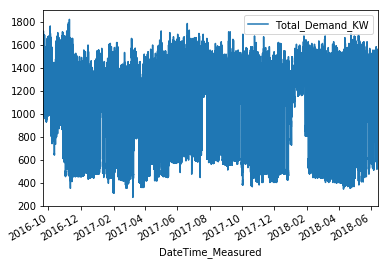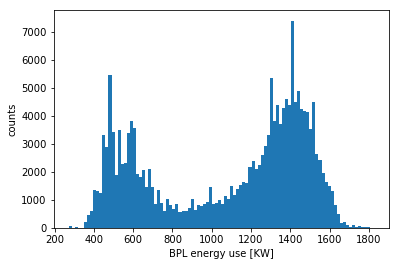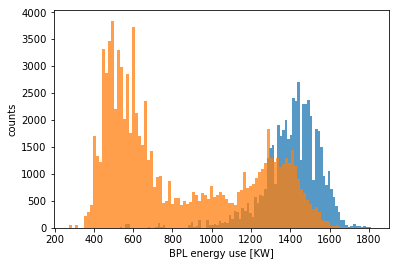# Testing notebooks with Boston Public Library energy data

I’d like to be able to display Jupyter notebooks as blog pages, so this will be a test of that idea. Below is a Jupyter notebook playing with the energy usage data from the Boston Public Library. To get the images to show up I need to write out the full path. There is probably a way to do this that involves less messy directory structure …

To do the conversion I used:

`````` jupyter nbconvert BPL_power.ipynb --to markdown
``````

Boston Public Library

I found the city of boston analytics department database, and it has all sorts of cool stuff. Let’s just load the BPL energy use and plot it, just to test things out, and just because I like libraries!

``````import pandas as pd
import matplotlib.pyplot as plt

%matplotlib inline
``````

``````BPL = pd.read_csv('https://data.boston.gov/dataset/652762e9-2556-47cd-8e80-798546992a57/resource/87c759ee-63b9-4aec-a00b-bba1672a20ef/download/copleylibraryelectricuse.csv', parse_dates=True, index_col= )

``````
``````                     Total_Demand_KW
DateTime_Measured
2018-06-14 12:25:00           1555.0
2018-06-14 12:20:00           1555.0
2018-06-14 12:15:00           1483.0
2018-06-14 12:10:00           1519.0
2018-06-14 12:05:00           1519.0
``````
``````BPL.plot()

# plt.xlim(['June 1 2018', 'June 10 2018'])
``````
``````<matplotlib.axes._subplots.AxesSubplot at 0x11cf0af98>
``````Take a look at the distribution of power useage

``````plt.hist(BPL.Total_Demand_KW, 100);
plt.xlabel('BPL energy use [KW]')
plt.ylabel('counts')
``````
``````<matplotlib.text.Text at 0x1217d4f60>
``````Looks like the power usage is pretty bimodal. Probably the peak around 600 KW is when the library is closed, and when everything is open the library uses about 1400 KW. Weekdays the library is open from 9 am to 9 pm. Weekends it closes at 5. Let’s split the data roughly into open and closed and plot those histograms

``````BPL['day_of_week'] = BPL.index.weekday

# just get the open hours M-Th:
open_hours = BPL[BPL.day_of_week<=3].between_time('09:00', '21:00' )

# closed for sure after 9
closed_hours = BPL.between_time('21:00', '09:00' )
# you are still missing the weekend open hours! and a few closed hours between 5 and 9 pm
``````
``````# plt.hist(BPL.Total_Demand_KW, 100);
plt.hist(open_hours.Total_Demand_KW, 100, alpha=0.75)
plt.hist(closed_hours.Total_Demand_KW, 100, alpha=0.75)
plt.xlabel('BPL energy use [KW]')
plt.ylabel('counts')
``````
``````<matplotlib.text.Text at 0x11d731b70>
``````It does look like the peaks line up with the open and closed times. But there are still a lot of high usage values when the library is closed. We should check what this is about!

``````
``````

Updated: